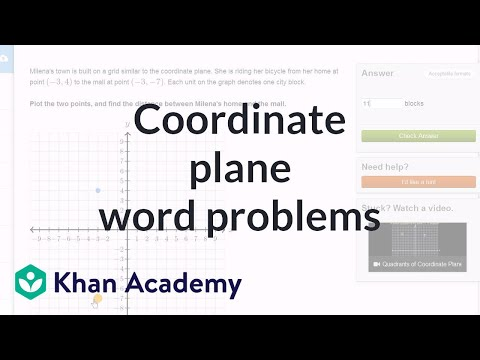Video

# Parallelogram on the coordinate plane (Full video)

Description: Remember our discussion of the coordinate plane? Sure you do! Let's graph the given coordinates of three of the polygon vertices, and find where the 4th vertex is. Created by Sal Khan. Vertex C is at the point 4.5 comma 4, so 4.5 along the horizontal axis comma 4. That right over there is point C. Point D is at negative 1.5 comma 4, so negative 1.5 along the horizontal or the x-axis, we could say, negative 1.5 comma 4, so 4 along the vertical or the y-axis. And we need to figure out what are the coordinates of point B if B must be in quadrant I.

### Other videos you might be interested in### Area of a parallelogram on the coordinate plane (Full video)